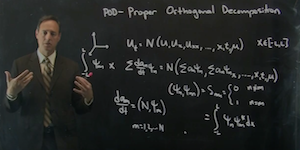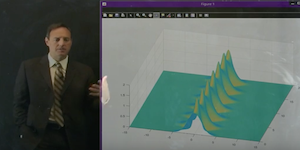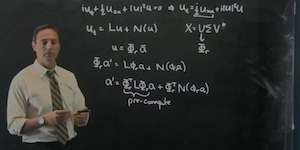# Reduced Order Modeling

## The POD method and Galerkin approximations

### Lecture 1### [ view ]

BASIS SELECTION AND IDEAL MODES: This lecture provides an introduction to basis representations, POD modes, and orthogonality of function spaces.

MATLAB COMMANDS

`FFT`

### Lecture 2### [ view ]

SPECIAL FUNCTIONS + GALERKIN PROJECTIONS: The harmonic oscillator is considered along with its ideal basis functions: the Gauss-Hermite polynomials.

MATLAB COMMANDS

`SVD`

### Lecture 3### [ view ]

POD EXPANSION : We construct a ROM model of the nonlinear Schrodinger equation using a 2 POD mode expansion.

MATLAB COMMANDS

`ODE45`

MATLAB CODE